## A projectile’s horizontal range on level ground is R= [(Vo)^2 sin2θ] / g. At what launch angle or angles will the projectile land at half of

Question

A projectile’s horizontal range on level ground is R= [(Vo)^2 sin2θ] / g. At what launch angle or angles will the projectile land at half of its maximum possible range.

in progress 0
2 weeks 2021-08-30T03:35:32+00:00 1 Answers 0 views 0

15° and 75°

Explanation:

Given:

The range of a projectile motion is given as: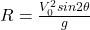Where,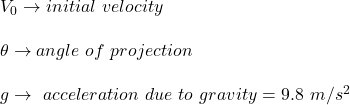Maximum possible range is when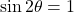So, maximum range possible is,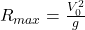Now, as per question: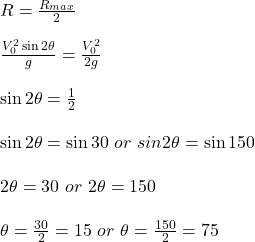Therefore, the launch angles are 15 degree and 75 degree.